## Regression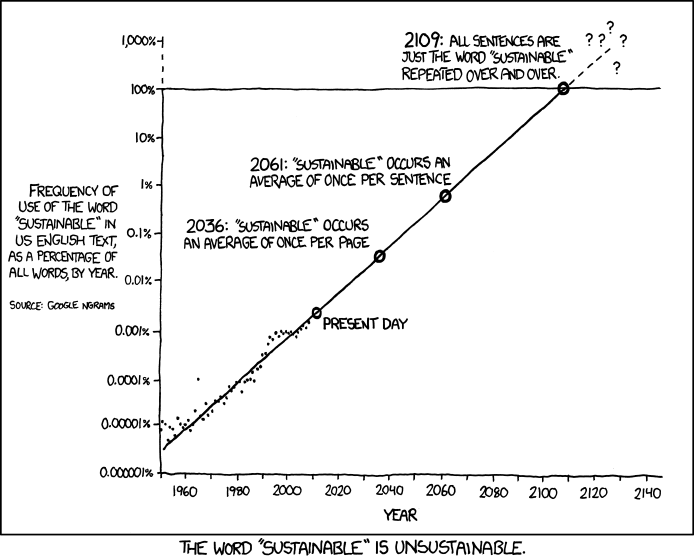## Correlation doesn't imply CausationBut it may, if there is a good independent reason to think that X influences Y.

## Regression implies X causes Y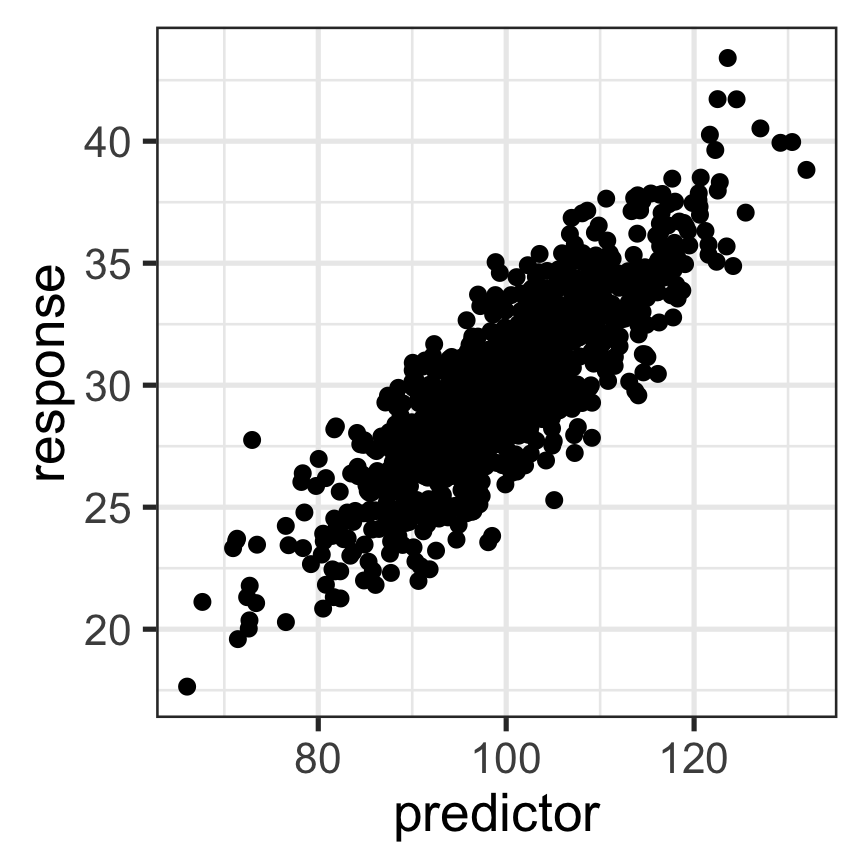$Y = slope \times X + intercept$

• Both variables are continuous
• TONS of data are suitable for this kind of analysis.
• Examples?

## Simple Linear Model

Simplest way two variables can be modeled as related to one another

$Y_i = \beta_0 + \beta_1X_i + \epsilon_i$

• $$\beta_0$$ is the intercept (value of y where x= 0)
• $$\beta_1X_i$$ is the slope value expressing $$\Delta Y / \Delta X$$
• $$\epsilon_i$$ is the error term
• normal random variable with mean 0 and variance $$\sigma^2$$

## This equation should make sense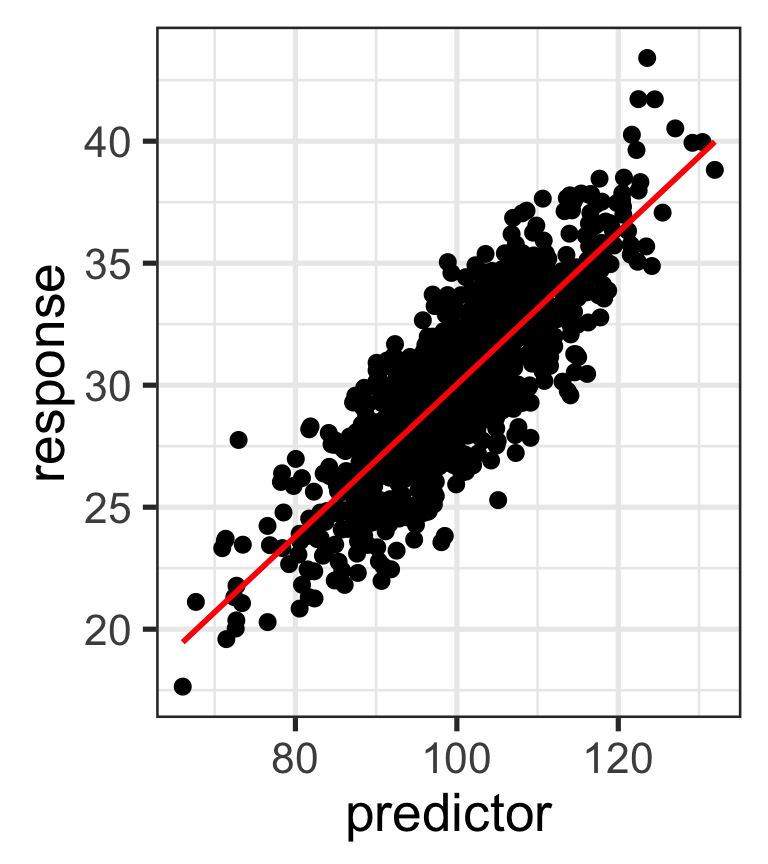$Y_i = \beta_0 + \beta_1X_i + \epsilon_i$

Once you decide on a line, then the value of Y equals:

• the value predicted by the line, plus
• a random error from our error term

## Finding the best line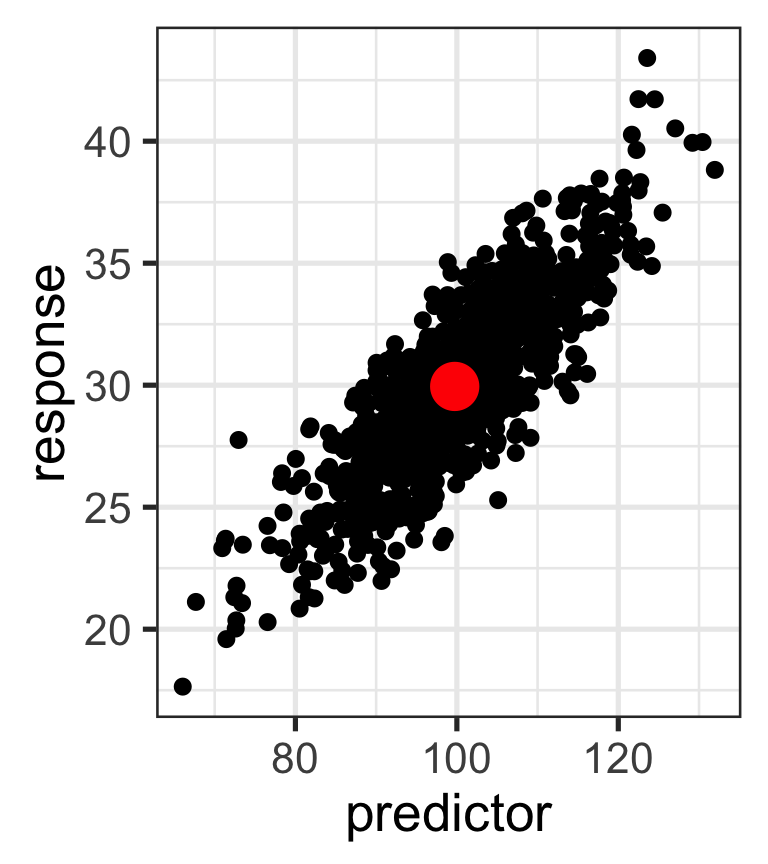• Once we decide on linear regression, we have to find best line
• There is unexplained variation in Y, so points don't fall on straight line (why?)
• Many lines pass through $$(\bar{X},\bar{Y})$$. How do we pick the best one?

## Finding the best line - residuals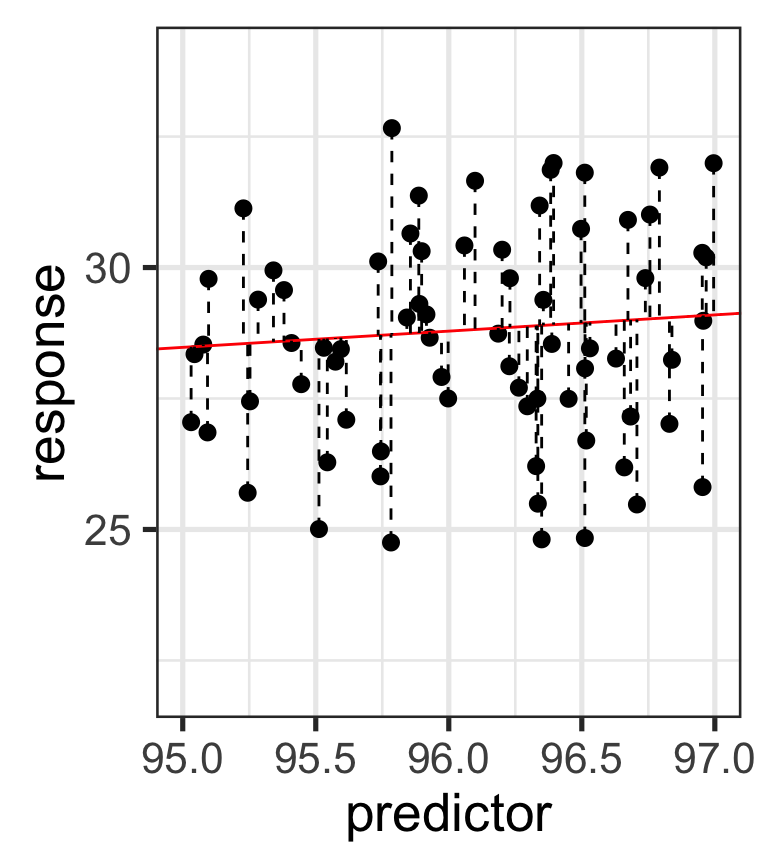A residual represents the distance between the predicted value from the regression, and the actual value.

Another way to think about the residual: a single value from the normally distributed error term.

The squared residual is calculated as such:

$d_i^2=(Y_i - \hat{Y}_i)^2$

## Finding the best line

The best line minimizes the residual sum of squares

$RSS=\sum\limits_{i=1}^n(Y_i - \hat{Y}_i)^2$

We could do a Monte Carlo approach: try a bunch of slopes passing through $$(\bar{X},\bar{Y})$$ and calculate the RSS, then pick the smallest, but math offers a simpler solution.

## Variances and Covariances

Recall the sum of squares: $\ SS_Y = \sum\limits_{i=1}^n(Y_i - \bar{Y})^2$

Sample variance: $\ s^2_Y=\frac{\sum\limits_{i=1}^n(Y_i - \bar{Y})^2}{n-1}$

SS equivalent to: $\ SS_Y = \sum\limits_{i=1}^n(Y_i - \bar{Y})(Y_i - \bar{Y})$

## Variances and Covariances

With 2 variables, we can define sum of cross products $\ SS_{XY} = \sum\limits_{i=1}^n(X_i - \bar{X})(Y_i - \bar{Y})$

By analogy to the sample variance, we define sample covariance $\ s_{XY} = \frac{\sum\limits_{i=1}^n(X_i - \bar{X})(Y_i - \bar{Y})}{(n-1)}$

## Sample Covariance - Negative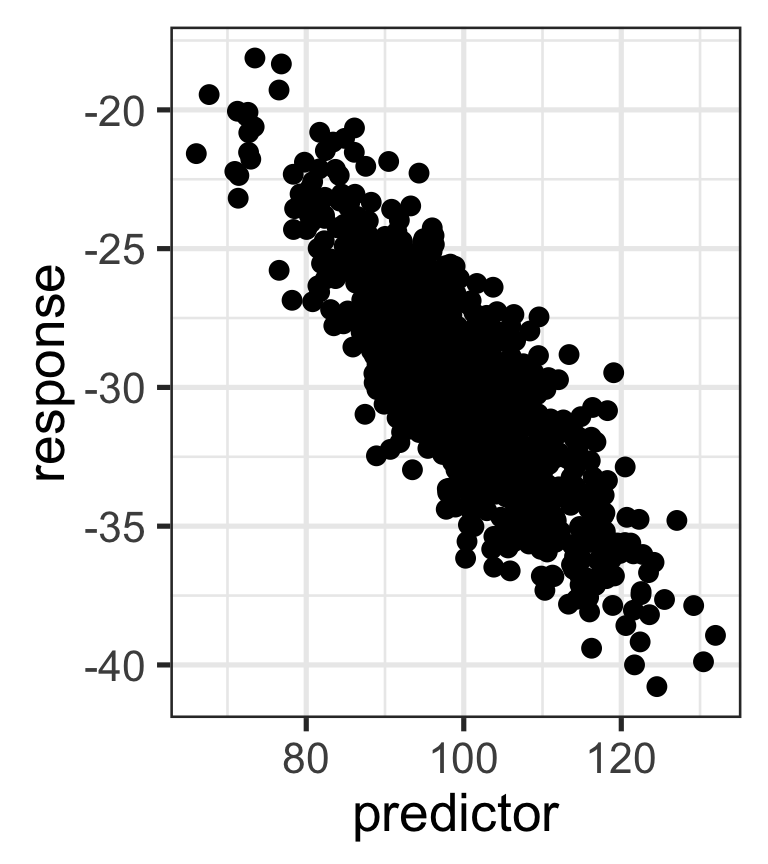From $$-\infty$$ to $$\infty$$

$\ s_{XY} = \frac{\sum\limits_{i=1}^n(X_i - \bar{X})(Y_i - \bar{Y})}{(n-1)}$